Subsections

# Quantum registers

## Structures

All quantum computation is done with quantum registers. libquantum provides a structure for a quantum register:

 quantum_reg {    int width; /* number of qubits in the register */    int size; /* number of non-zero vectors */    int hashw; /* width of the hash array */    quantum_reg_node *node;    int *hash; };

To achieve an efficient memory usage, the quantum register only contains the basis vectors with a non-zero probability amplitude. All these basis states are coded in an array of the following type:

 quantum_reg_node {    COMPLEX_FLOAT amplitude;    MAX_UNSIGNED state; };

This implementation is equivalent to the widely used description of a quantum state vector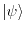with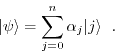In this case, amplitude is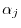and state is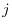.

## quantum_new_qureg

 extern quantum_reg quantum_new_qureg(MAX_UNSIGNED initval, int width);

This function is used to create a quantum register. initval contains the value to which the register is initialized at the beginning. On most machines, it is a 64-bit integer. width contains the number of qubits the register should start with. Note that the size of the hash table is computed according to this value, so additional scratch space should be added later with quantum_addscratch.

## quantum_new_qureg_size

 extern quantum_reg quantum_new_qureg_size(int n, int width);

This function creates an empty quantum register with n basis states for width qubits. Use this function only if you want to do time evolution. Filling each node with values lies within your responsibility.

## quantum_delete_qureg

 extern void quantum_delete_qureg(quantum_reg *reg);

Deletes a quantum register and frees its allocated memory.

## quantum_print_qureg

 extern void quantum_print_qureg(quantum_reg reg);

Prints the contents of a quantum register to the standard output stream.

 extern void quantum_addscratch(int bits, quantum_reg *reg);

This function adds bits of scratch space to a quantum register. The scratch space is initialized to zero and gets inserted at the least significant bit of the register, i.e. the register changes from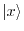to. Note that this space is not included for computing the size of the hash table, so creating new basis states in the scratch space will overfill the hash table.

## quantum_kronecker

 extern quantum_reg quantum_kronecker(quantum_reg *reg1, quantum_reg *reg2);

This function creates the Kronecker product of two registers. The target register is linked with the control registers by the following scheme:

Suppose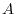is a-lined vector andis a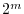-lined vector, then we have the representation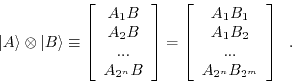## quantum_dot_product

 COMPLEX_FLOAT quantum_dot_product(quantum_reg *reg1, quantum_reg *reg2);

Computes the dot product of two quantum registers. Let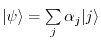anddenote the two quantum registers reg1 and reg2, respectively, then the dot product is